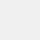# Camera math calculator

نسخهٔ ۶.۰.۵.۳۸۵
+۲ هزار
نصب
ابزارها
دسته‌بندی
۱۷ مگابایت
حجم# Camera math calculator

Scientific calculator developer
نسخهٔ ۶.۰.۵.۳۸۵
+۲ هزار
نصب
ابزارها
دسته‌بندی
۱۷ مگابایت
حجم

## معرفی برنامه

جزئیات بیشتر

Math calculator solves maths problem by taking photo!

Math calculator is a useful and advanced calculator pro with basic calculator free, equation solver and scientific calculator. This stylish calculator is also an equation solver and a great maths homework solver which can solve maths problems more efficiently by CAMERA .

Let's see what's inside:
➡️ Maths Camera: Good maths homework helper which solves maths problems by taking photo.
➡️ Advanced scientific Calculator: Multifunctional and scientific.
➡️ Equation calculator: Solve your maths homework efficiently.

👍 Math calculator camera - photo calculator
We provide a mathematics solutions in a creative way. While you are doing maths home work, try our calculator so that you can solve maths problems more easily by taking photo and get homework answers quickly. It's a homework solver. Both basic maths problem and equations are suitable.

👍 Scientific Calculator Plus
Scientific calculator is an advanced calculator for student and engineering. Advanced calculator pro can calculate everything from basic math such as pre-algebra to advanced calculation calculus. It's an easy-to-use calculator. This scientific calculator contains all scientific calculations such as trigonometric, power, logarithmic, square root, cube root, factorial, π calculation.

👍 Equation calculator
There is a equation solver designed for students who need to solve equations and inequalities. What’s more, you can do decimal calculation and power calculation in the equation calculator.

نظرات کاربران - ۱۰ رأی
۴ از ۵
۵
۴
۳
۲
۱zahra
۱۴۰۰/۰۸/۱۵
خیلی خوبه من از این استفاده زیادی میکنم عالی دست سازنده درد نکتهسینا
۱۴۰۰/۱۲/۱۰
سلام‌ کَسی می دونه چطور از دوربین این برنامه می شه استفاده کرد ؟nouri
۱۴۰۰/۱۱/۲۰
عالی